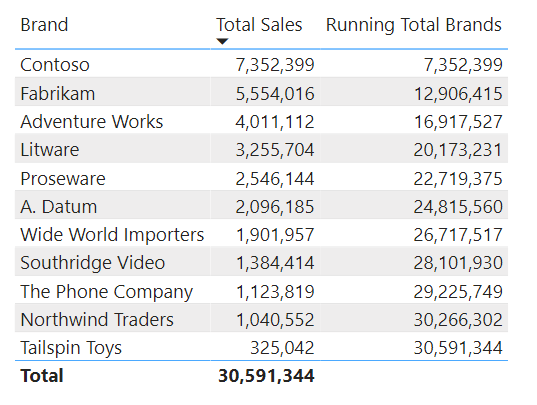top of page
Search
•Antriksh Sharma

# How to create Running Total on Non-Numeric Fields in Power BI using DAX

Updated: May 18, 2021

Creating running totals on a numeric or date field is pretty easy because you always have something that you can use to compare against the lower or higher value.

With Integers you can simply calculate numers of items that are less than or greater than the current item using COUNTROWS and FILTER construct or by using RANKX and for dates we have Time Intelligence functions such as DATESYTD, DATESBETWEEN etc.

But creating running totals on a non-numeric fields can prove to pretty challenging, so let's see two ways of calculting running totals on a text field such as Products[Brand]

So how can we calculate running totals on a report like the following one:?Let's see 2 ways of calculating Running Total

One way to do is by evaluating at each cell of the report, how many Total Sales are greater than the sales of that Brand:

First let's find out the Sales Amount of the Brand visible in the current filter contex and stored it in a variable:

Then create another variable that will store Sales Amount of all the brands in the model.

The above variable will create a temporary table in memory which will look like this:Being able to create virtual tables in memory using ADDCOLUMNS or GENERATE/ROW construct helps in creating complex code on fly and they sometimes help in writing neat and optimized code.

Moving on, now we will filter brands that have sales amount greater than equal to the sales amount of the current brand stored in the CurrentBrandSales variable

To create a rank we can use COUNTROWS:

Before computing the Running Total let's see the ranking that the code we have written so far is creating:Now that we have ranking all we need to do is compute the sales amount by creating a variable which is a simple SUMX over the previously computed variable.

This is the what the Result variable returns:The whole code now looks like this

Let's see another way of computing same running total but this time using RANKX only. Being able to write the same code in multiple ways helps in understanding DAX beter and you can even find a way to optimize your slow performing code.

This time we start by creating Rank using RANKX which returns the same rank that we saw using COUNTROWS, nothing special in this, only thing to pay attention to is that we are now getting a rank at the grand total as well, we will see later how we can remove that.

This is the ranking we get:Next we create a new measure and we need to declare a variable which will store the ranking of the current product visible in the filter context.

Next we need to create a temporary table that will contain the rank for each brand as well as the sales amount.

The contents of this variable will look something like this:Now we can use this in memory table to filter brands that have Rank less than or equal to the rank of the current brand:

The next thing is to compute the sales amount:

This is what the Result variable returns:Notice that we get some value at the grand total, we want to remove that how can we do it?

we can use ISINSCOPE to check if the Brand is currently being grouped by and as you may know at the grand total the grouping doesn't happens becuase at the grand total DAX engines calculate ROLLUPADDISSUBTOTAL or ROLLUPGROUP

This how the final version of the code looks like:

And now you can see that the ranking has been removed from the grand total: